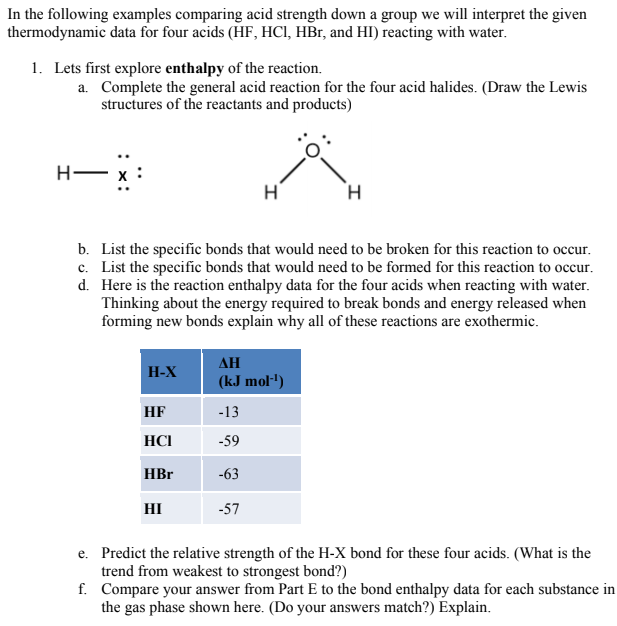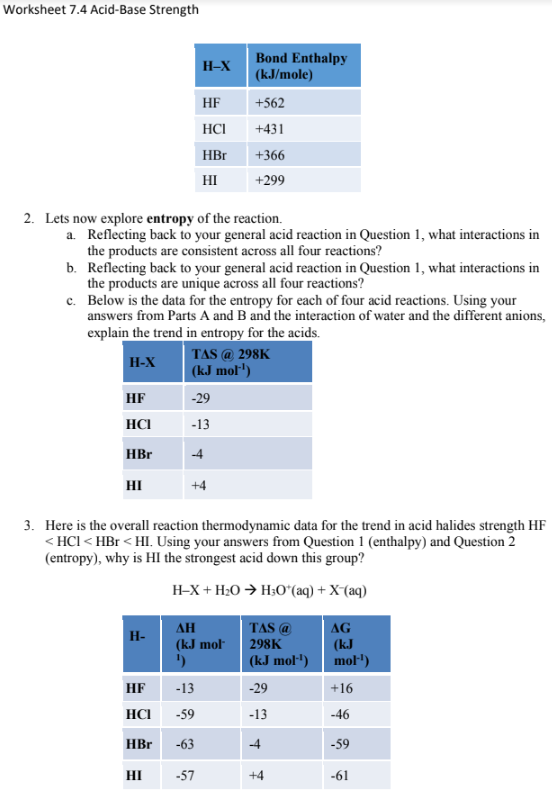# In the following examples comparing acid strength down a group we will interpret the given thermodynamic data for four acids (HF, HCl, HBr, and HI) reacting with water. 3. Here is the overall reaction thermodynamic data for the trend in acid halides strength HF < HCl < HBr < HI. Using your answers from Question 1 (enthalpy) and Question 2 (entropy), why is HI the strongest acid down this group? H–X + H2O → H3O+(aq) + X^–(aq)